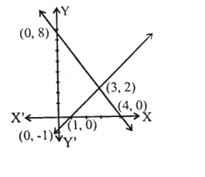# CREST Mathematics Olympiad Class 10 Sample Papers

## Syllabus:

Section 1: Real Numbers, Polynomials, Pair of Linear Equations in Two Variables, Quadratic Equations, Arithmetic Progressions, Triangles, Coordinate Geometry, Introduction to Trigonometry, Some Applications of Trigonometry, Circles, Constructions, Areas Related to Circles, Surface Areas and Volumes, Statistics, Probability.

Achievers Section: Higher Order Thinking Questions - Syllabus as per Section 1

 Q.1 What is the length of base of triangle formed by lines x – y – 1 = 0, 2x + y = 8 and y-axis in the figure below?Q.2 Which of the following equations has the sum of its roots as 3?
 Q.3 The value of [(cos A/cot A) + sin A] is:
 Q.4 The area of the circle that can be inscribed in a square of side 6 cm is:
 Q.5 For what value of k, do the equations 3x - y + 8 = 0 and 6x - ky = -16 represent coincident lines?
 Q.6 At one end A of a diameter AB of a circle of radius 5 cm, tangent XAY is drawn to the circle. The length of the chord CD parallel to XY and at a distance 8 cm from A is:
 Q.7 If the lines given by 3x + 2ky = 2 and 2x + 5y + 1 = 0 are parallel, then the value of k is:
 Q.8 The lengths of the diagonals of a rhombus are 16 cm and 12 cm. Then, the length of the side of the rhombus is:
 Q.9 What is the coefficient of x2 in the polynomial P(x) = 3x3 + 10(x-x2) -5x2 -2?
 Q.10 A boy divided the numbers 7654, 8506 and 9997 by a certain larger number and he gets the same remainder in each case. What is the common remainder?

Sample PDF of CREST Mathematics Olympiad for Class 10: